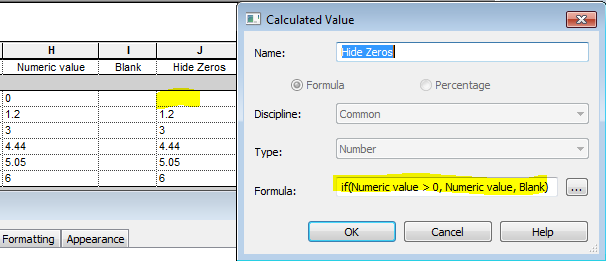# Hide Zero Values in Multiple Item Rows in Revit SchedulesHide zero values in schedules (Previous post)

Following on from a previous post about how to Hide Zero Values in Revit Schedules, I have noticed that it does not work when you untick ‘Itemise each Instance’ (Multiple items per row) and you set the formatting to calculate totals – the zero returns.

In the example above, you probably would want to show the zeros when calculating totals of different numbers – but there are situations where you legitimately do not want to show the zero.  Here follows an example, and yet another workaround to solve that problem.

### Example of Workflow

A situation where you may not want to show the zeros might be when the field is a count of items.  As demonstrated in my previous “Count Dracula” post, it is not possible to include the system parameter ‘Count’ in a Schedule formula; nor is it possible to make a ‘Count’ value of zero – the workaround to that problem is to create your own ‘MyCount’ parameter, as described…